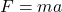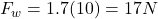Question

A hammer has a mass of 1.7kg. How much does it weigh?

1.thongdat2

17 N

Explanation:

Weight is a force. The equation to calculate a force is:where a is the acceleration of the object. On an object simply existing, the force of gravity adds an acceleration of 9.8m/s², or approximately 10m/s². So, to calculate the force of weight, we simply multiply the mass by 10 for that reason:Forces are measured in Newtons (N) so the answer is 17 N.